# Intermediate Algebra Tutorial 39

Intermediate Algebra

WTAMU > Virtual Math Lab > Intermediate AlgebraLearning Objectives

After completing this tutorial, you should be able to:
1. Multiply radicals that have the same index number.
2. Divide radicals that have the same index number.Introduction

In this tutorial we will be looking at rewriting and simplifying radical expressions.  Part of simplifying radicals is being able to take the root of an expression which is something that is shown in  Tutorial 37: Radicals.  It is good to be comfortable with radicals before entering College Algebra. I think you are ready to move ahead into the tutorial.Tutorial

With the Same Index Number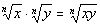In other words, when you are multiplying two radicals that have the same index number, you can write the product under the same radical with the common index number.

Note that if you have different index numbers, you CANNOT multiply them together.

Also, note that you can use this rule in either direction depending on what your problem is asking you to do.Example 1: Use the product rule to multiply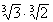.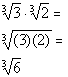*Use the prod. rule of radicals to rewrite

Note that both radicals have an index number of 3, so we were able to put their product together under one radical keeping the 3 as its index number.

Since we cannot take the cube root of 6 and 6 does not have any factors we can take the cube root of, this is as simplified as it gets.Example 2: Use the product rule to multiply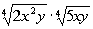.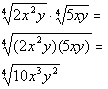*Use the prod. rule of radicals to rewrite

Note that both radicals have an index number of 4, so we were able to put their product together under one radical keeping the 4 as its index number.

Since we cannot take the fourth root of what's inside the radical sign and 10 does not have any factors we can take the fourth root of, this is as simplified as it gets.

With the Same Index Number

If n is even, x and y represent any nonnegative real number
and y does not equal 0.

If n is odd, x and y represent any real number and y does not equal 0.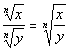This works in the same fashion as the rule for a product of two radicals.

This rule can also work in either direction.Example 3: Use the quotient rule to simplify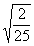.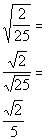*Use the  quotient rule of radicals to rewrite

*Square root of 25 is 5

Since we cannot take the square root of 2 and 2 does not have any factors that we can take the square root of, this is as simplified as it gets.Example 4: Use the quotient rule to simplify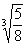.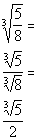*Use the  quotient rule of radicals to rewrite

*The cube root of 8 is 2

Since we cannot take the cube root of 5 and 5 does not have any factors that we can take the cube root of, this is as simplified as it gets.

When you simplify a radical, you want to take out as much as possible.

We can use the product rule of radicals in reverse to help us simplify the nth root of a number that we cannot take the nth root of as is, but has a factor that we can take the nth root of.  If there is such a factor, we write the radicand as the product of that factor times the appropriate number and proceed.

We can also use the quotient rule to simplify a fraction that we have under the radical.

Note that the phrase "perfect square" means that you can take the square root of it.  Just as "perfect cube" means we can take the cube root of the number, and so forth.  I will be using that phrase in some of the following examples.Example 5: Simplify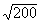.

Even though 200 is not a perfect square, it does have a factor that we can take the square root of.

Check it out: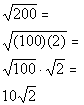*Rewrite 200 as (100)(2)

*Use the prod. rule of radicals to rewrite
*The square root of 100 is 10

In this example, we are using the product rule of radicals in reverse to help us simplify the square root of 200.  When you simplify a radical, you want to take out as much as possible.  The factor of 200 that we can take the square root of is 100.  We can write 200 as (100)(2) and then use the product rule of radicals to separate the two numbers.  We can take the square root of the 100 which is 10, but we will have to leave the 2 under the square root.Example 6: Simplify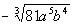.

Even though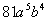is not a perfect cube, it does have a factor that we can take the cube root of.

Check it out: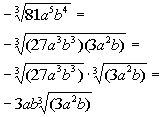*Rewriteas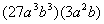*Use the prod. rule of radicals to rewrite
*The cube root of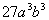is 3ab

In this example, we are using the product rule of radicals in reverse to help us simplify the cube root of.  When you simplify a radical, you want to take out as much as possible.  The factor ofthat we can take the cube root of is.  We can writeasand then use the product rule of radicals to separate the two numbers.  We can take the cube root of, which is 3ab, but we will have to leave the rest of it under the cube root.Example 7: Use the quotient rule to divide and then simplify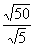.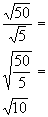*Use the  quotient rule of radicals to rewrite

*Simplify fraction

Note that both radicals have an index number of 2, so we are able to put their quotient together under one radical keeping the 2 as its index number.

50/5 simplifies to be 10.  Since we cannot take the square root of 10 and 10 does not have any factors we can take the square root of, this is as simplified as it gets.Example 8: Use the quotient rule to divide and then simplify.  Assume that the variables are positive.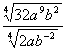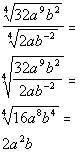*Use the  quotient rule of radicals to rewrite

*Simplify fraction
*Take the fourth root

Note that both radicals have an index number of 4, so we are able to put their quotient together under one radical keeping the 4 as its index number.

Since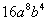is a perfect fourth, we are able to take the fourth root of the whole radicand, which leaves us with nothing under the radical sign.Practice Problems

These are practice problems to help bring you to the next level.  It will allow you to check and see if you have an understanding of these types of problems. Math works just like anything else, if you want to get good at it, then you need to practice it.  Even the best athletes and musicians had help along the way and lots of practice, practice, practice, to get good at their sport or instrument.  In fact there is no such thing as too much practice.

To get the most out of these, you should work the problem out on your own and then check your answer by clicking on the link for the answer/discussion for that  problem.  At the link you will find the answer as well as any steps that went into finding that answer.Practice Problem 1a: Use the product rule to multiply.

1a.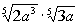Practice Problem 2a: Use the quotient rule to simplify.

2a.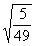Practice Problems 3a - 3b: Simplify.  Assume that the variables are positive.

3a.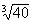3b.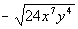Practice Problem 4a: Use the quotient rule to divide and the then simplify.
Assume that the variables are positive.

4a.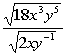Need Extra Help on these Topics?

Go to Get Help Outside the Classroom found in Tutorial 1: How to Succeed in a Math Class for some more suggestions.Last revised on July 20, 2011 by Kim Seward.